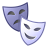# QlikView App Dev

Discussion Board for collaboration related to QlikView App Development.

Announcements
cancel
Showing results for
Did you mean:Creator III

## Set analysis sum between two given dates

Hello,

i want to calculate sum between two given dates using set analysis. I am want to use greater than or equal to startdate and less than or equal to Enddate.

I am using this formula. but its not working even for 1 condition. How to use less than or equal to condition in ths formula??/

sum({\$<OrderDate>={StartDate}>} Netamountpaid )

thanks

Lavi

1 Solution

Accepted SolutionsMVP

Hi Lavi,

venkatreddy's solution will work, but only if StartDate and EndDate are limited to one value in current selection.

For example, if a user selects none or more than one Date, the expression will not work.

You could combine the expression with a dollar sign expansion to handle date ranges:

= sum({<OrderDate= {">=\$(=min(StartDate))<=\$(=max(EndDate))"}> } Netamountpaid)

I assume you are not using StartDate and EndDate as dimensions in a table, with above expression, else you are running into troubles, since the set analysis will not care about the dimensions.

Regards,

Stefan

13 RepliesPartner

Hi, you need to add this into the set expression writing something like this:

sum({\$<OrderDate={'<=\$(=only(StartDate))'}>} Netamountpaid )

Thanks

SteveNot applicable

Using following expression

sum({\$<OrderDate={">=StartDate<=Enddate"}>Netamountpaid)Creator III
Author

Steve,

thanks for the response. in your formula what is StartDate??

is it variable or field name.

if it is variable then where to define it?

in my formula StartDate is field name

Regards

HarleenCreator III
Author

thanks for the response. in your formula what is StartDate??

is it variable or field name.

if it is variable then where to define it?

in my formula StartDate is field name

Regards

HarleenNot applicable

field NamePartner

Hi, yes i was also assuming it was a field name

Thanks

SteveMVP

Hi Lavi,

venkatreddy's solution will work, but only if StartDate and EndDate are limited to one value in current selection.

For example, if a user selects none or more than one Date, the expression will not work.

You could combine the expression with a dollar sign expansion to handle date ranges:

= sum({<OrderDate= {">=\$(=min(StartDate))<=\$(=max(EndDate))"}> } Netamountpaid)

I assume you are not using StartDate and EndDate as dimensions in a table, with above expression, else you are running into troubles, since the set analysis will not care about the dimensions.

Regards,

StefanCreator II

Hi Stefan,

Will the same expression works for current year and last year data as well, i tried below expression

For current year

Sum({<Year = {\$(=max(Year))},Date={">=\$(=min(Promo_Date_From))<=\$(=max(Promo_Date_To))"}>} [Net Sales])

here am usning promo event as dimension.

it's giving 0 as output. Please suggest.

Regards,Contributor III

Hi Stefan ,

i m using a set analysis expression . in my report i m showing data for LMTD.  In Dimension i have LinkCommonDate and used: